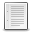File : Details

# pdiv_inc()

Polynomial division with increasing terms powers
Details
Version
2.0
Author
Samuel Gougeon
Maintainer
Samuel Gougeon
Category
Supported Scilab Version
5.5
Creation Date
August 27, 2015Description
```            --> pdiv_inc

Polynomial division with increasing terms powers

SYNTAX
------
pdiv_inc   // Displays this help
[q, r] = pdiv_inc(p, d)
[q, r] = pdiv_inc(f)

PARAMETERS
----------
p,d,q,r: Single polynomials with real or complex coefficients.
p : polynomial to be divided
d : divisor. Its first non-null coefficient of lowest power must
be of power equal or lower than p's one.
q : quotient, with deg(q) <= deg(p)
r : remainder, with deg(r) <= deg(p)+deg(d)
such that: p = q*d + r. p and d are not necessarily coprime.

f : Single rational, with coprime polynomials p = numer(f) and d = denom(f)

DESCRIPTION
-----------
pdiv_inc(p, d) performs the Euclidian division p/d with polynomial terms
of p and d sorted by increasing powers. It returns the quotient q and the
remainder r, such that p = q*d + r.

Detailled example:
2 - x + 3x^2               | 1 + 2x^2
---------------------------|------------
2     + 4x^2               | 2 - x - x^2
- x -  x^2               |
- x        - 2x^3        |
-  x^2 + 2x^3        |
-  x^2        - 2x^4 |
2x^3 + 2x^4 |
Hence, (2 - x + 3x^2) = (2 - x - x^2)*(1 + 2x^2) +  (2x^3 + 2x^4)

NOTES:
* If d==0, q=0 and r=p are returned whatever is p.
* if both p and d are limited to a decimal, there are set to p+0*x and d+0*x

WARNING: If f is specified explicitly through a p/d ratio with p and d not
coprime, their common factors are first simplified, and the p = q*d + r
relationshift holds only with simplified p => sp and d => sd.
Example: p = poly([1 2 2 3 3],'x'), d = poly([2 3 4],'x'), p/d
[q,r] = pdiv_inc(p/d), p-q*d-r, f = p/d; numer(f)-q*denom(f)-r

REFERENCE
---------
Comments, scoring and bug reports are welcome on
http://fileexchange.scilab.org/toolboxes/449000#new_comment

--------
pdiv   : Polynomial division by decreasing terms powers
modulo : Remainder after polynomial division
ldiv   : Limited Taylor series of a polynomial ratio

EXAMPLES
--------
x = poly(0,"x");
// 1) Simple example
p =  1 + 3*x + 2*x^2 - 7*x^3
d = -1 +   x - 2*x^2
[q,r] = pdiv_inc(p,d),  p-q*d-r   // => 0

// 2) With some non-leading null coefficients in p and/or d
p = 1 + x +      3*x^3
d = 1 +    2*x^2
[q,r] = pdiv_inc(p,d),  p-q*d-r   // => 0

// 3) With p not starting with x^0
p =    x -   x^2 + 2*x^3
d = -1   + 2*x^2
[q,r] = pdiv_inc(p,d),  p-q*d-r   // => 0

// 4) With p and d not starting with x^0
p =       x^2 + 2*x^3
d =  -x + 2*x^2 - x^3
[q,r] = pdiv_inc(p,d),  p-q*d-r   // => 0

// 5) with complex coefficients:
p = -1 + (1+%i)*x - %i*x^2 ;
d = %i - x + (1-%i)*x^2    ;
[q,r] = pdiv_inc(p,d);
sci2exp(q), sci2exp(r), sci2exp(p-q*d-r)   // => 0

// 6) d must start at a degree lower or equal to p's starting degree
p =  2 -  x + 3*x^2
d =     2*x -   x^2
[q,r] = pdiv_inc(p,d)  //=> Error

// 7) Random example
p = poly(grand(1,5,"uin",-9,9), "x", "coeff")
d = poly(grand(1,7,"uin",-9,9), "x", "coeff")
[q,r] = pdiv_inc(p,d),  clean(p-q*d-r)   // => 0
```Files (2)Screenshot
```Screenshot of an example for thumbnail
```Miscellaneous file
```File defining the function pdiv_inc(), help contents and examples

* MAKE pdiv_inc() AVAILABLE in EVERY SESSION: Click here to see HOW TO

* Get help: Enter "pdiv_inc" without parameters

CHANGES 1.0 => 2.0
------------------
* Minor improvements in the help
```News (0)Comments (0)